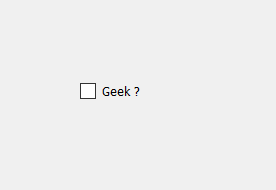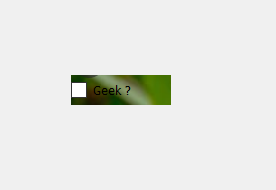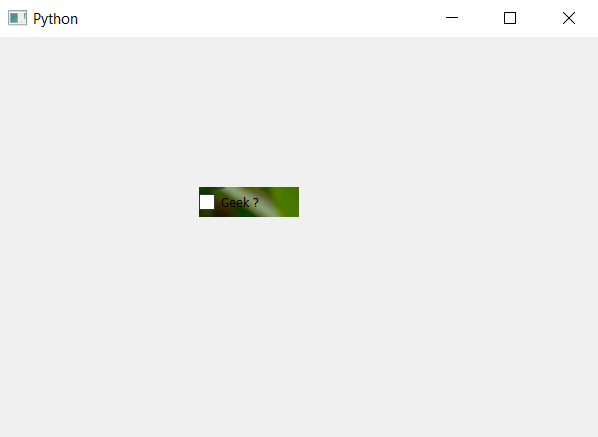Open In App

# PyQt5 – Image in Check Box

In this article we will see how to set background image to check box. When we create a check box there is no image associated to it although we can set background color to it. Below is the representation of normal check box vs the check box which has image.In order to do this we have to set background image in style sheet, below is the style sheet code which can be used with the check box object.

```QCheckBox
{
background-image : url(image.png);
}
```

Below is the implementation.

 `# importing libraries``from` `PyQt5.QtWidgets ``import` `*` `from` `PyQt5 ``import` `QtCore, QtGui``from` `PyQt5.QtGui ``import` `*` `from` `PyQt5.QtCore ``import` `*` `import` `sys`` ` ` ` `class` `Window(QMainWindow):`` ` `    ``def` `__init__(``self``):``        ``super``().__init__()`` ` `        ``# setting title``        ``self``.setWindowTitle(``"Python "``)`` ` `        ``# setting geometry``        ``self``.setGeometry(``100``, ``100``, ``600``, ``400``)`` ` `        ``# calling method``        ``self``.UiComponents()`` ` `        ``# showing all the widgets``        ``self``.show()`` ` `    ``# method for widgets``    ``def` `UiComponents(``self``):`` ` `        ``# creating the check-box``        ``checkbox ``=` `QCheckBox(``'Geek ?'``, ``self``)`` ` `        ``# setting geometry of check box``        ``checkbox.setGeometry(``200``, ``150``, ``100``, ``30``)`` ` `        ``# setting stylesheet``        ``# adding background image to check box``        ``checkbox.setStyleSheet(``"QCheckBox"``                               ``"{"``                               ``"background-image : url(image.png)"``                               ``"}"``)`` ` ` ` ` ` `# create pyqt5 app``App ``=` `QApplication(sys.argv)`` ` `# create the instance of our Window``window ``=` `Window()`` ` `# start the app``sys.exit(App.``exec``())`

Output :